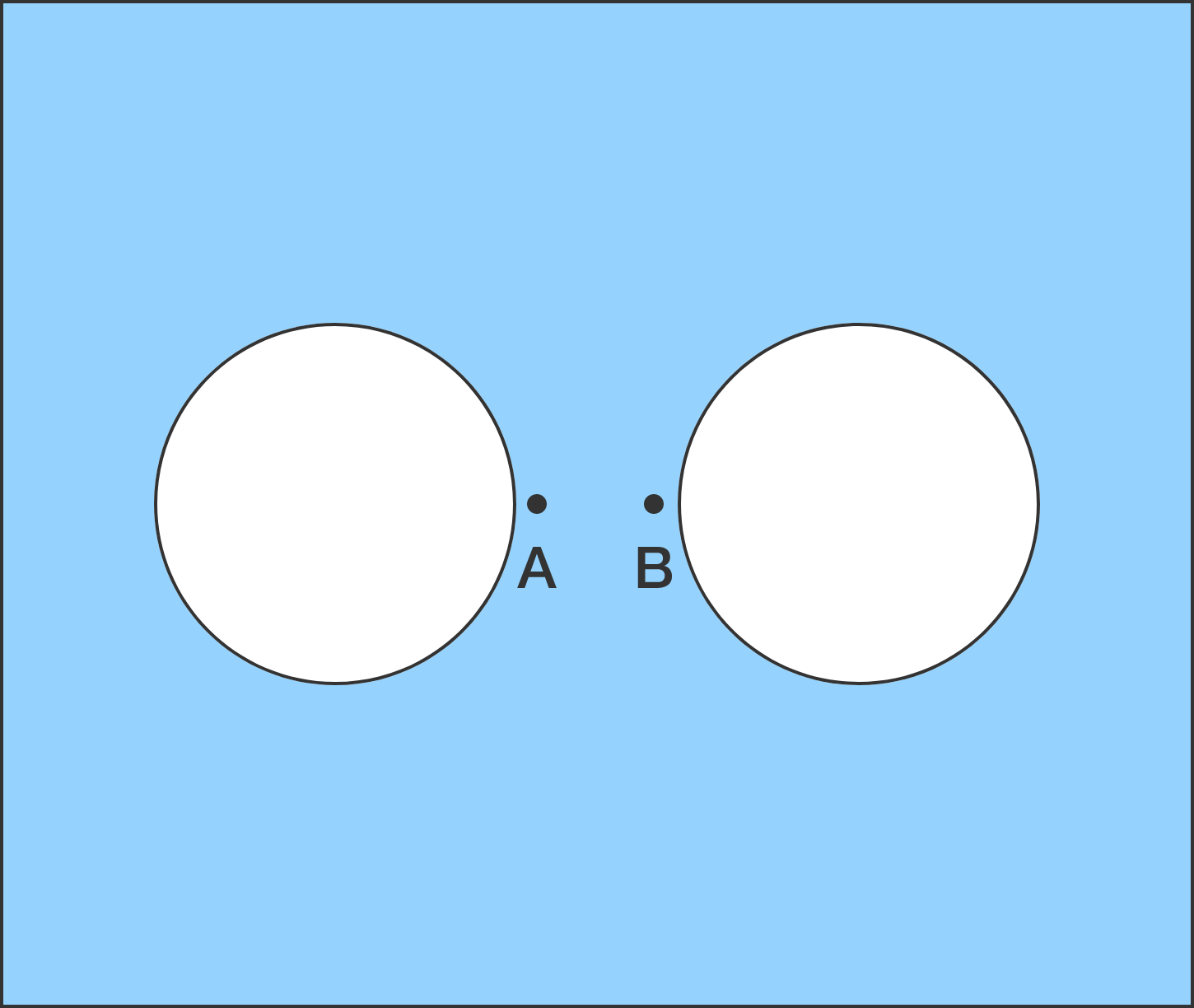# Thermal expansion

Two identical circular holes are made in a metallic square sheet, and two tiny points $A$ and $B$ are marked next to the holes, as shown in the diagram.

If the temperature of the whole sheet is uniformly raised, then the size of the holes will $\text{\_\_\_\_\_\_\_\_\_\_}$ and the distance between $A$ and $B$ will $\text{\_\_\_\_\_\_\_\_\_\_}.$×